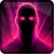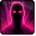# Zweiter AuftragConditions

## Used by

Triggered by equipping an item (1)

## Related effects, buffs and debuffs

Please click on an effect below to view its details.

• [hidden] [does not expire]Effect #1

 Slot: EffectOther Duration: passive Tick rate: does not tick # occurrences: 1
• On Apply

Only when the following conditions are met:

• If TARGET is one of these classes:
- 16141170711935532310

Perform the following actions:

• Modify meta stat #422, fixed = 1
• On Apply

Only when the following conditions are met:

• If TARGET is one of these classes:
- 16140973599688231714

Perform the following actions:

• Modify meta stat #422, fixed = 1
• After an ability is activated by NONE
• After an ability is activated by NONE

Perform the following actions:

• Add effect #2 to TARGET from TARGET
• Just before this effect is removed

Only when the following conditions are met:

• Unknown (91)
- Negated = (bool) false
- Tag = (string)
- Actor = (int) 3
- Ability Spec = (int) 16141048995493192440
- Comparison = (int) 6
- Amount = (float) 2

Perform the following actions:

• Unknown (193)
- Unknown (601) = (bool) false
- Unknown (602) = (bool) false
- Unknown (607) = (bool) false
- Tags = (string)
- Ability Spec = (int) 16141048995493192440
- Amount Max = (float) -1
- Amount Min = (float) -1
- Amount Percent = (float) 0
• Just before this effect is removed

Only when the following conditions are met:

• Unknown (91)
- Negated = (bool) false
- Tag = (string)
- Actor = (int) 3
- Ability Spec = (int) 16140981924952695063
- Comparison = (int) 6
- Amount = (float) 2

Perform the following actions:

• Unknown (193)
- Unknown (601) = (bool) false
- Unknown (602) = (bool) false
- Unknown (607) = (bool) false
- Tags = (string)
- Ability Spec = (int) 16140981924952695063
- Amount Max = (float) -1
- Amount Min = (float) -1
- Amount Percent = (float) 0
• [0s] [not displayed]Effect #2

 Slot: Buff Duration: 0 Sek. Tick rate: does not tick # occurrences: 0 Conditions: Can only by called by other effects
• On Apply

Perform the following actions:

• Modify Cooldown
- Is Percentage = (bool) true
- Tag = (string)
- Tags = (string)
- Ability Spec = (int) 16141084896297597523
- Amount Min = (float) 0
- Amount = (float) -1
• Modify Cooldown
- Is Percentage = (bool) true
- Tag = (string)
- Tags = (string)
- Ability Spec = (int) 16141063035537871253
- Amount Min = (float) 0
- Amount = (float) -1
• Modify Cooldown
- Is Percentage = (bool) true
- Tag = (string)
- Tags = (string)
- Ability Spec = (int) 16141123883341215421
- Amount Min = (float) 0
- Amount = (float) -1
• Modify Cooldown
- Is Percentage = (bool) true
- Tag = (string)
- Tags = (string)
- Ability Spec = (int) 16140987780396566767
- Amount Min = (float) 0
- Amount = (float) -1